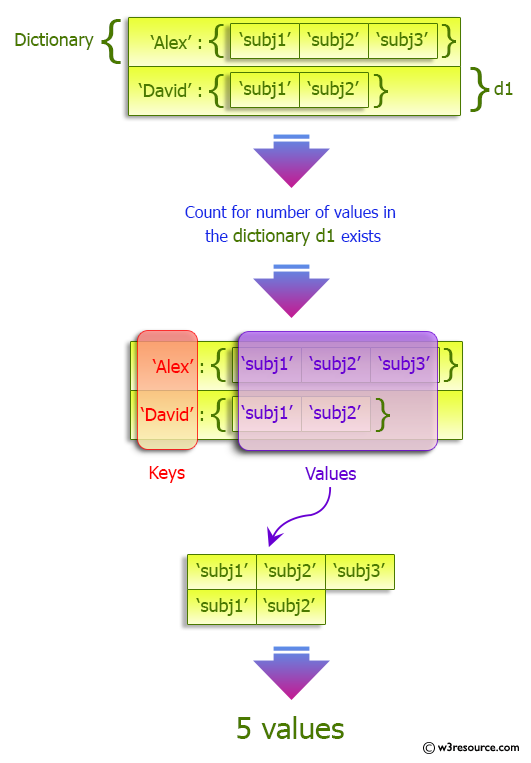﻿ Python: Count number of items in a dictionary value that is a list - w3resource# Python: Count number of items in a dictionary value that is a list

## Python dictionary: Exercise-34 with Solution

Write a Python program to count number of items in a dictionary value that is a list.

Sample Solution:-

Python Code:

``````dict =  {'Alex': ['subj1', 'subj2', 'subj3'], 'David': ['subj1', 'subj2']}
ctr = sum(map(len, dict.values()))
print(ctr)
```
```

Sample Output:

```5
```

Pictorial Presentation:## Visualize Python code execution:

The following tool visualize what the computer is doing step-by-step as it executes the said program:

Python Code Editor:

Have another way to solve this solution? Contribute your code (and comments) through Disqus.

What is the difficulty level of this exercise?

Test your Programming skills with w3resource's quiz.

﻿

## Python: Tips of the Day

Invokes the provided function after ms milliseconds:

Example:

```from time import sleep

def tips_delay(fn, ms, *args):
sleep(ms / 1000)
return fn(*args)
print(tips_delay(
lambda x: print(x),
1000,
'w3r'
))
```

Output:

```w3r
None
```